• 1. 学习使用Verilog HDL语言进行时序电路的设计方法 2. 掌握灵活运用Verilog HDL语言进行行为级语言描述的技巧和方法; 3. 学习寄存器堆的数据传送和读写工作原理,掌握寄存器堆的设计方法.
• 寄存器实验详解（寄存、移位）（74LS373、74LS299、74LS138、74LS245）含原工程文件
 寄存器实验实验要求分析设计具体实现（一）验证寄存器写操作（二）验证寄存器读操作（三）移位操作（四）循环移位操作思考问题
寄存器实验

先放一张 Proteus 总体仿真图，设计过程还是比较复杂的，需要考虑总体的布局，线路的排布等等。。。
我将原工程文件放在文末，需要可自取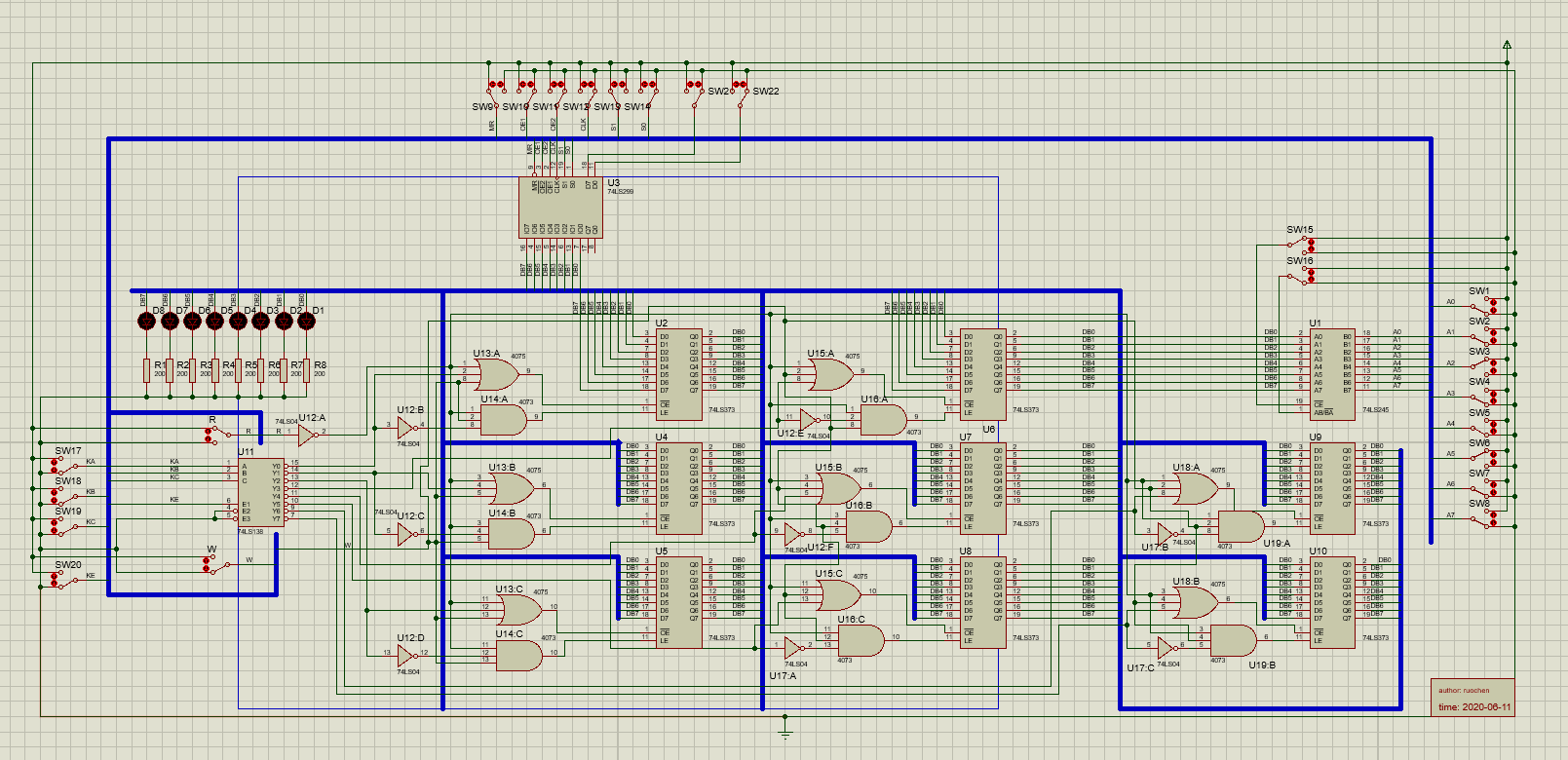实验要求

基本要求

理解CPU运算器中寄存器的作用
设计并验证寄存器组（至少四个寄存器）
利用寄存器或组合逻辑电路实现移位运算功能（至少含左移、右移、循环左移、循环右移四种运算功能）

扩展要求

实现更多的寄存器
实现多总线结构寄存器访问

分析

实验要求设计验证寄存器，还有实现移位功能，扩展功能是使用多总线结构和更多寄存器，这里我使用双总线结构，八个寄存器。

实验中用到的元器件如下

74LS373（寄存器）
74LS299（通用移位/存储寄存器）
74LS138（译码器，用来控制八个寄存器）
74LS245（数据传送）
SW-SPDT（单刀双掷开关）
LED（用来显示输出）
RES（LED下拉电阻）
4073（与门）
4075（或门）
74LS04（非门）

74LS299 功能表如下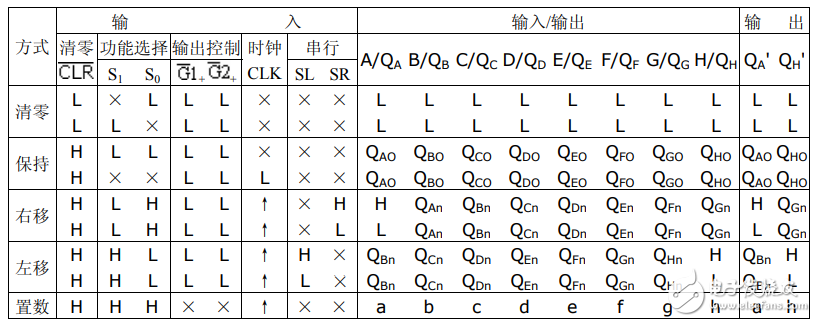其功能被表引脚与Proteus元器件有些许不同

CLR 对应 MR（低电平有效）

不管任何状态，强制清零

输出控制 G1、G2对应 OE1、OE2（低电平有效）

需要输出时，将这两个引脚置0

CLK

脉冲信号
查看功能表即可，需要上升沿时，就将开关拨高再拨低

D7、D0

下一位移位的数

Q7、Q0

保存最高位和最低位

其他元器件的使用自行百度即可

设计

这里说一下怎么使用 74LS138 和门电路组合实现控制八个 74LS373

我们使用 74LS138 的输出和 两个开关（一个读开关、一个写开关）作为输入，因为我们实现能够 同时控制 74SL373 的读/写
对应的输出就是 74LS373 的读和写
可得到如下真值表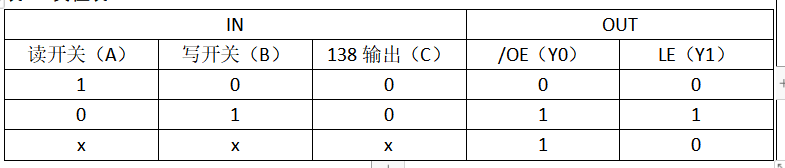对应表达式如下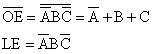最后根据表达式进行连线即可

运行流程图如下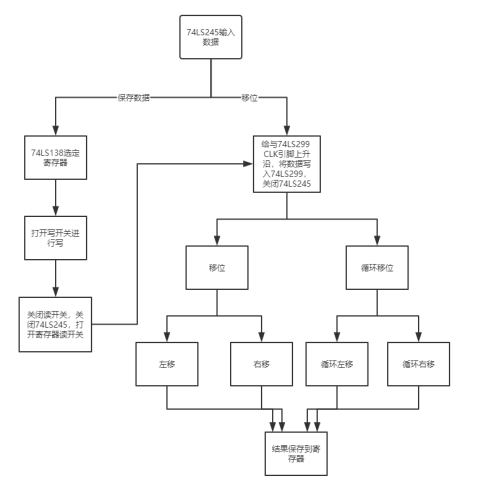电路设计可根据下述操作慢慢理解

具体实现
（一）验证寄存器写操作

首先，通过开关给 74LS245 输入数据，打开 74LS245 开关（CE引脚置0），将数据传送到总线上。如果需要对数据进行保存，通过 74LS138 输入开关（A、B、C）选通某个 74LS373 寄存器，打开 74LS373 的写开关（OE引脚），将数据写入 74SL373 ，然后关闭写开关，关闭 74LS245（CE引脚置1）。关闭 74LS245 后，此时总线上无数据。(放一张中间的图吧，下图对应的 74LS138 选通的是第一个寄存器，打开 74SL373 写开关)

我这里测试的八位数据是 00100100，选择的寄存器是第一个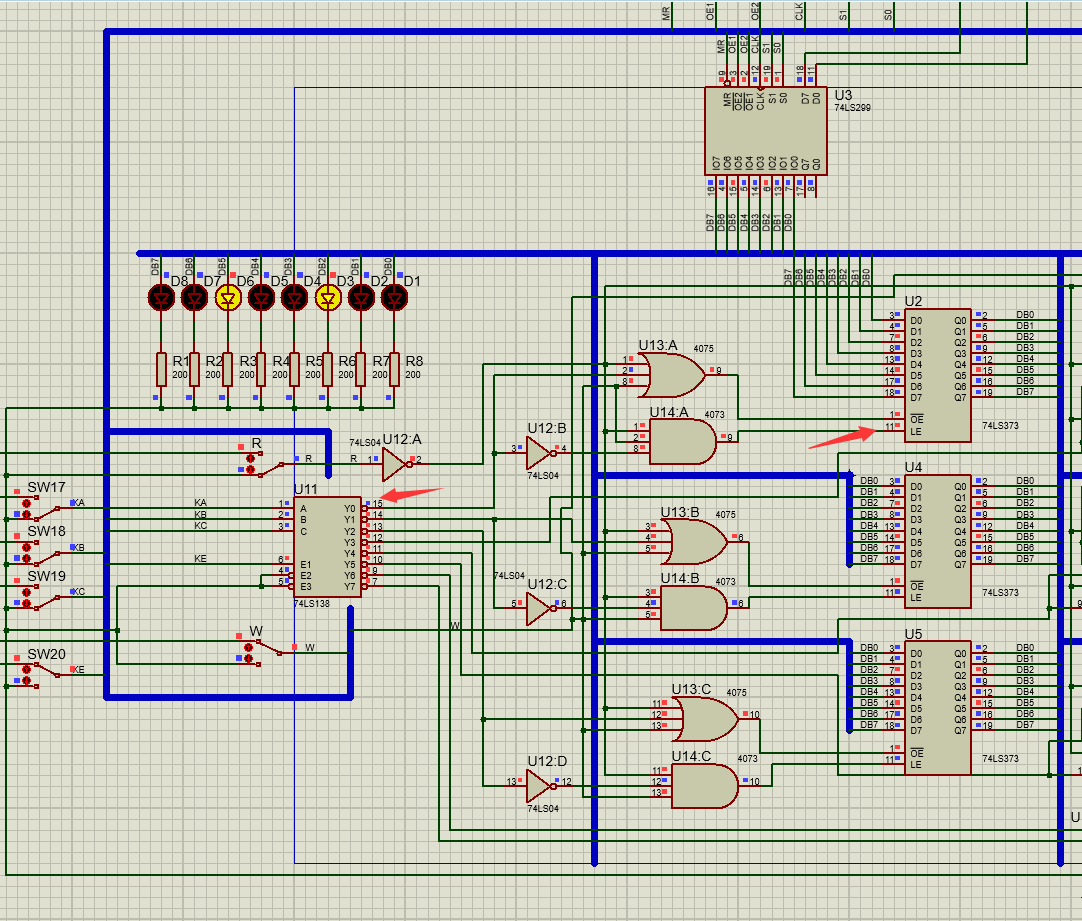（二）验证寄存器读操作

接着上一步，将 74LS373 的读开关（LE引脚）打开，将 74LS373 中所存的数据输出到总线上，读操作完成。
如图，此时 74LS245 是关闭的（CE引脚为1）的，打开 74LS373 的读开关后，数据输出到总线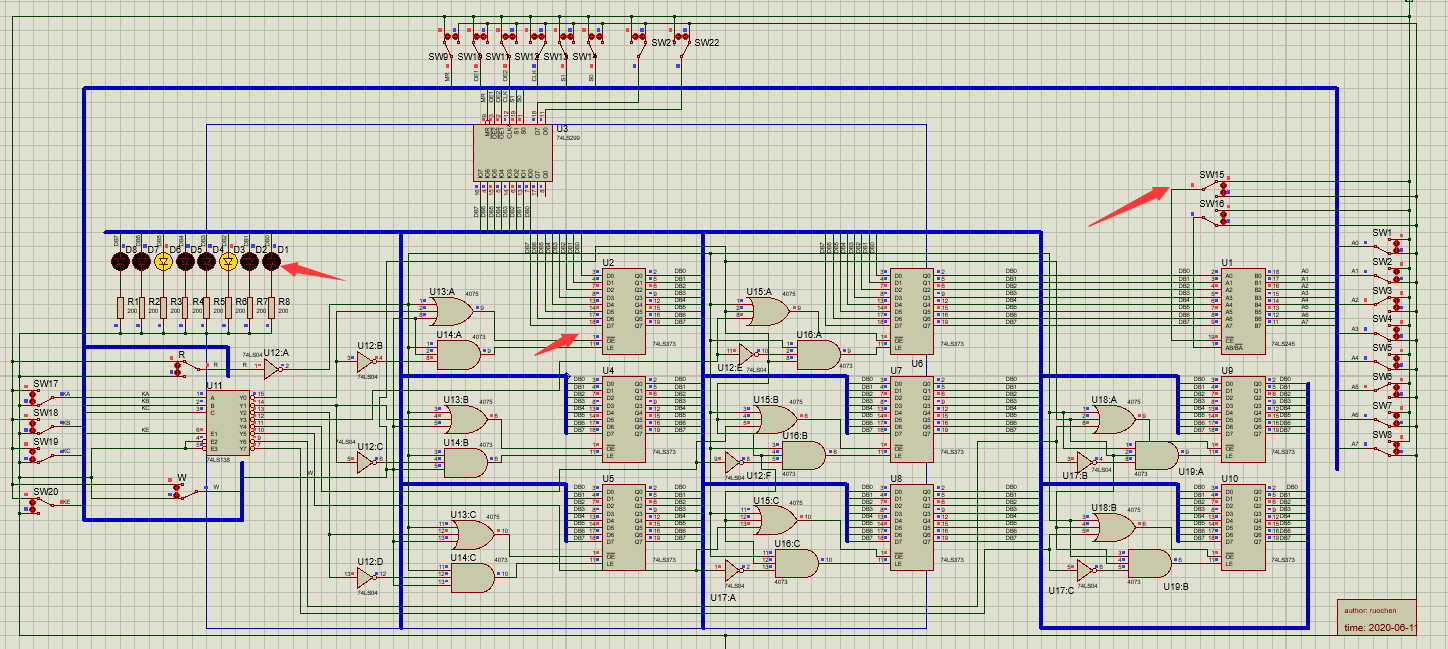（三）移位操作

移位和循环移位的区别

循环移位区别于一般移位的是移位是 没有数位的丢失。
循环左移时,用从左边移出的位填充字的右端,而循环右移时,用从右边移出的位填充字的左侧
例：   10110011

右移1位得到   ：01011001
循环右移1位得到：11011001

进行移位操作前，需要先将总线上的数据写入 74LS299 ，接着上一步，我们已经将 74LS373 的数据输出到总线上，然后我们给与 74LS299 的 CLK 引脚一个上升沿，就可以将总线上的数据写入 到74LS299 中， 然后关闭 74LS373 的读开关（此时总线无数据）

左移操作

74LS299 的 OE1、OE2、S1 、D7、D0 引脚均置 0，S0、MR 引脚置1（MR为0强制清零），持续给 CLK  上升沿就可以了（将开关重复拨上拨下） ，观察 LED 的变化。
如下图，持续进行左移操作，发现 最高位被移出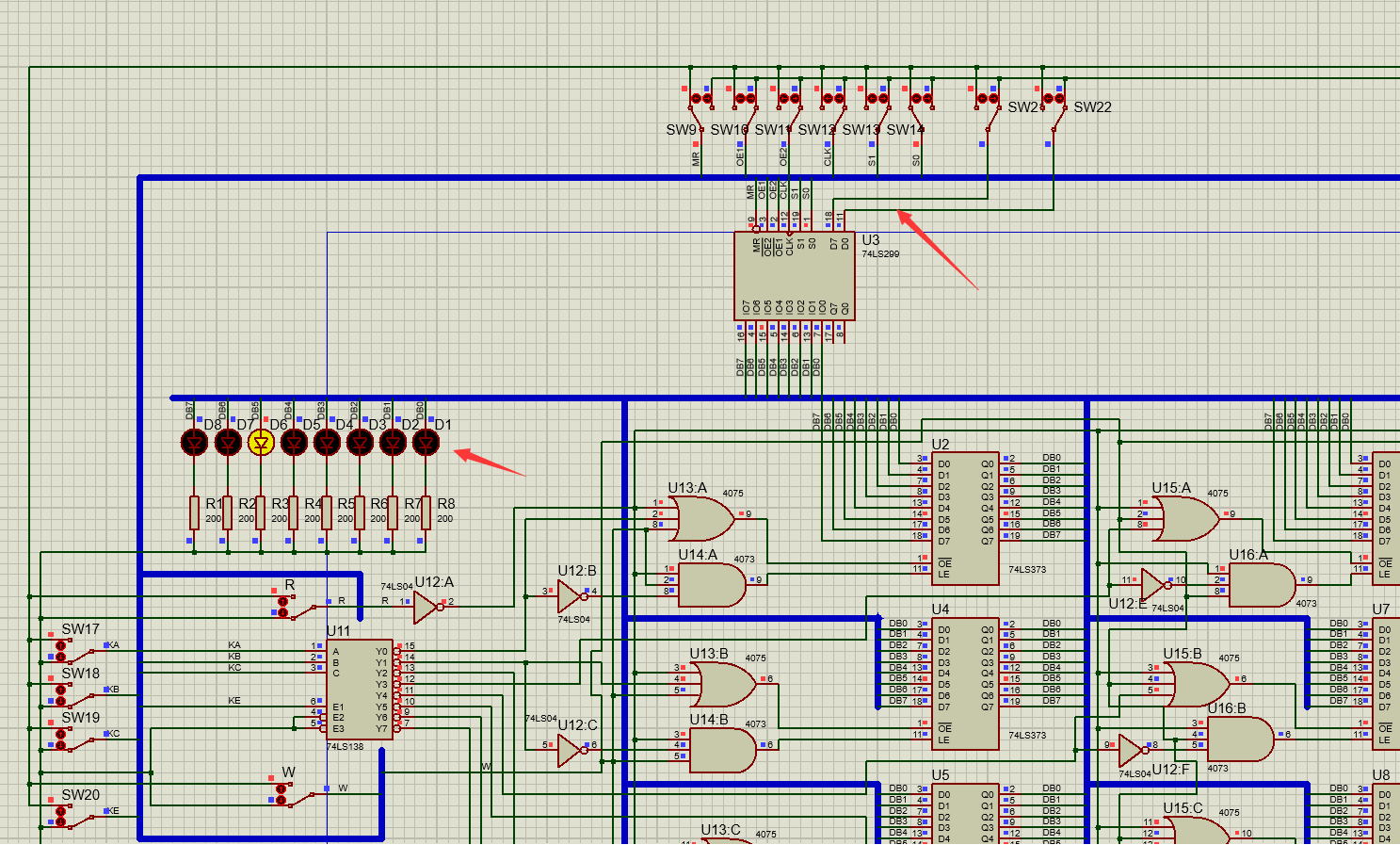右移操作

右移 S1置1，S0 置0，OE1、OE2、S0 、D7、D0 引脚均置 0，S1、MR 引脚置1，同样持续给与 CLK 引脚上升沿，然后观察 LED 的变化。
如下图，持续进行右移操作，发现 最低位被移出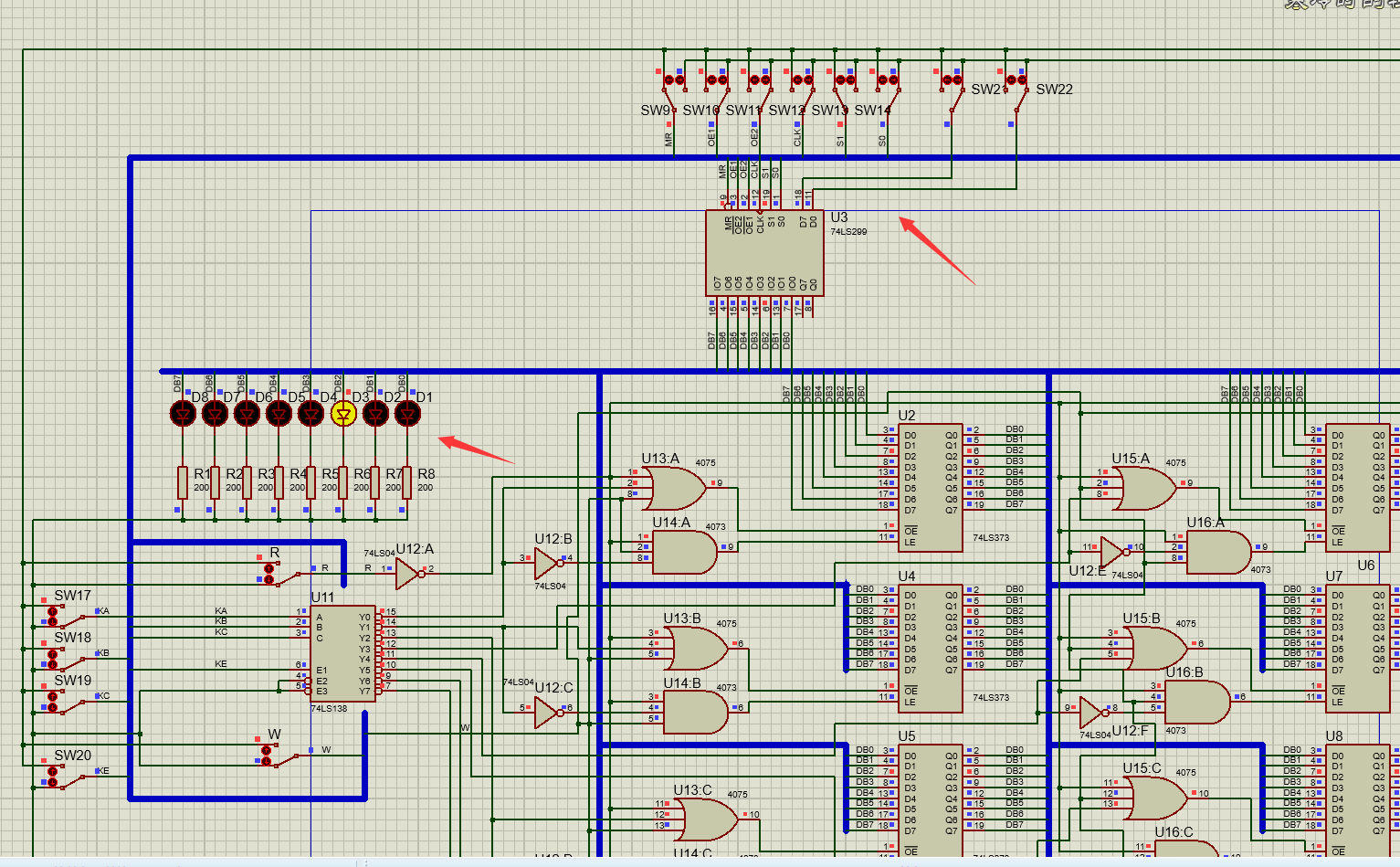（四）循环移位操作

循环左移

将 74LS299 的 D7 引脚接到Q0引脚，D0 引脚接到 Q7 引脚，OE1、OE2、S1 、D7、D0 引脚均置 0，S0、MR 引脚置1，持续给与 CLK 上升沿，观察 LED 变化。
如下图进行循环左移操作，我们可以看出，循环左移最高位移动到了最低位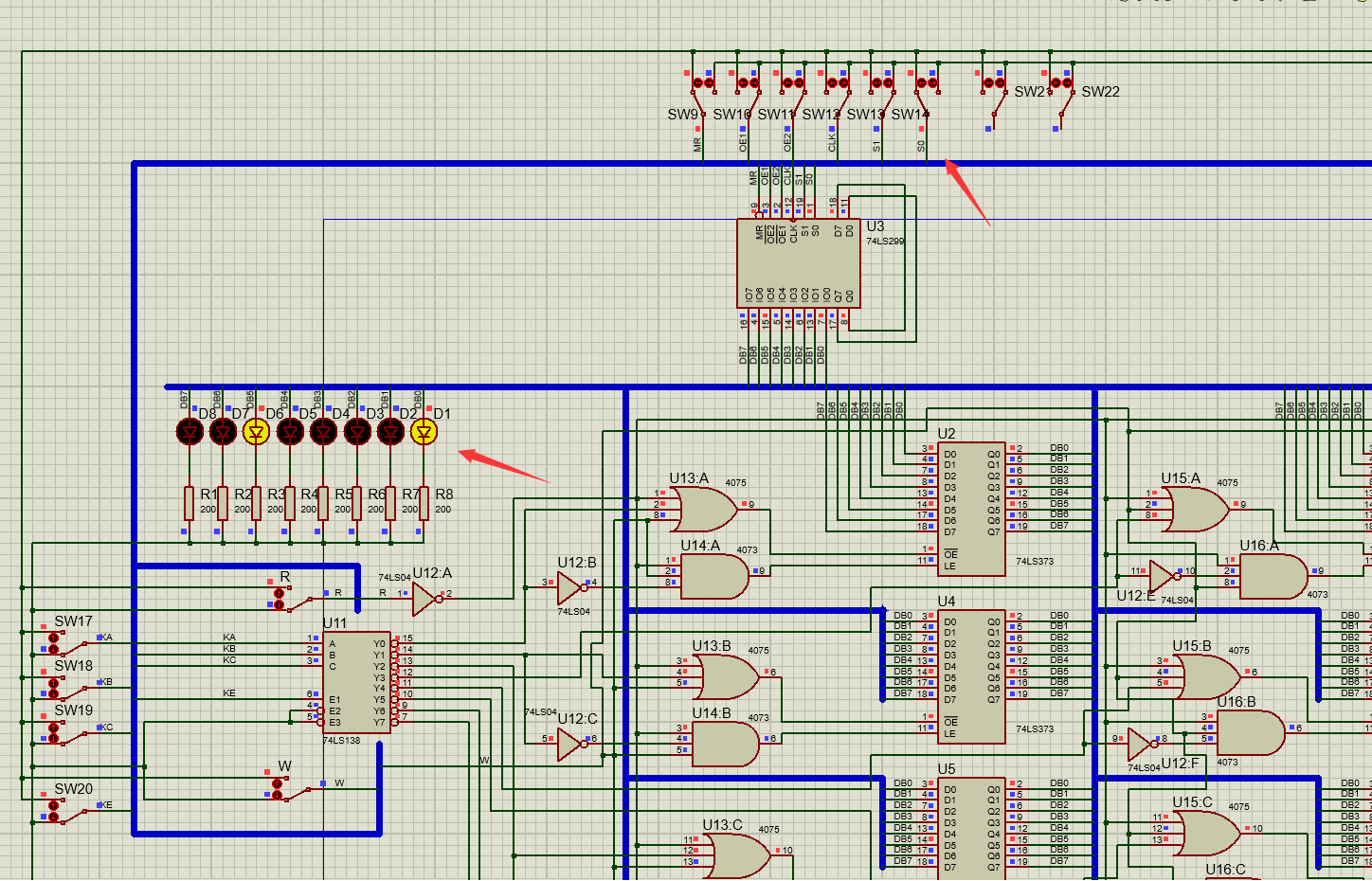循环右移

将 74LS299 的 D7 引脚接到Q0引脚，D0 引脚接到 Q7 引脚，OE1、OE2、S0 、D7、D0 引脚均置 0，S1、MR 引脚置1，持续给与 CLK 上升沿，观察 LED 变化。

如下图进行循环右移操作，我们可以看出，循环右移最低位移动到了最高位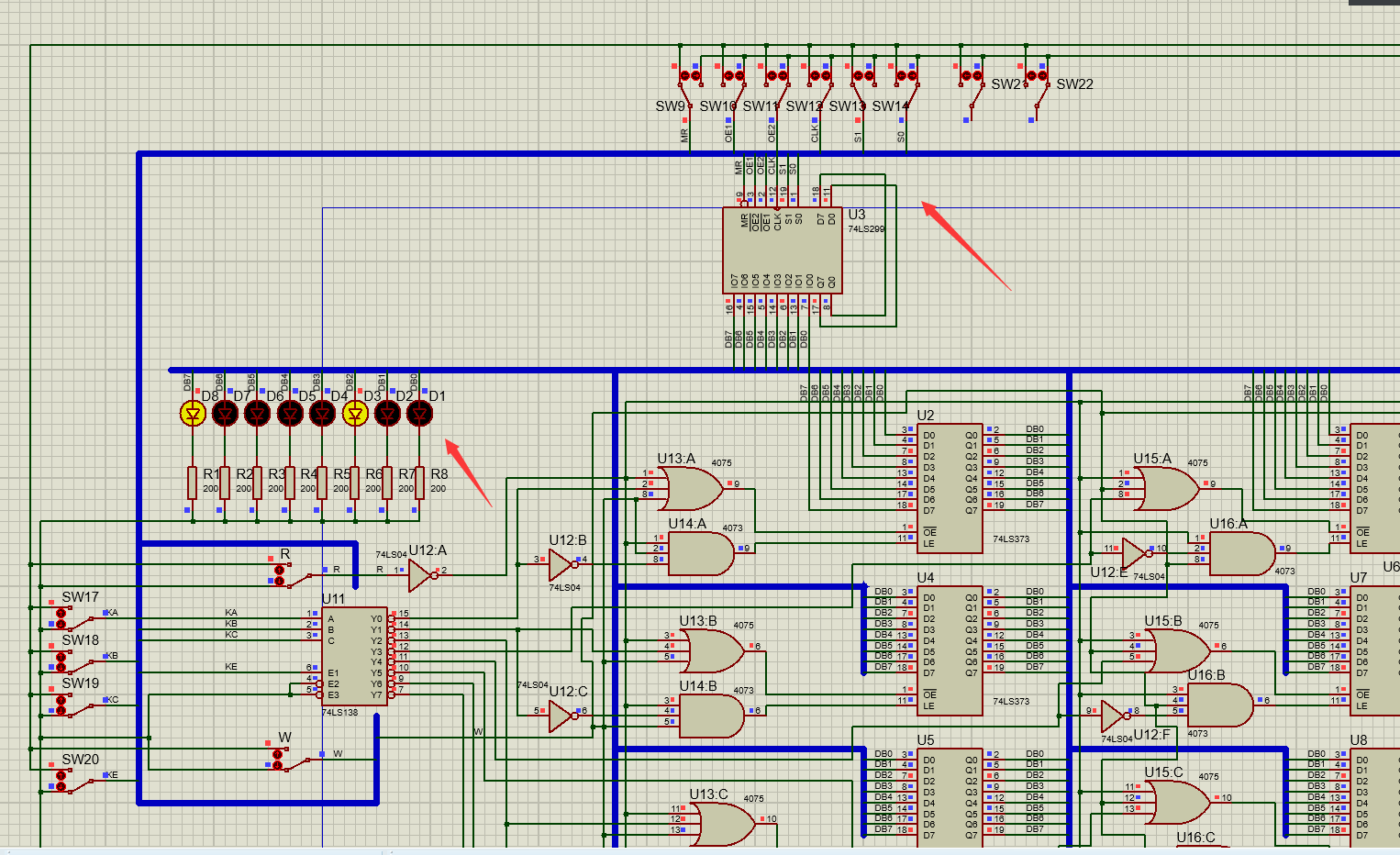我们可以将循环右移后的总线上的数据写入 74LS373 中。如图，将总线上的数据写入到 74SL373 中，并进行了 读操作（LE）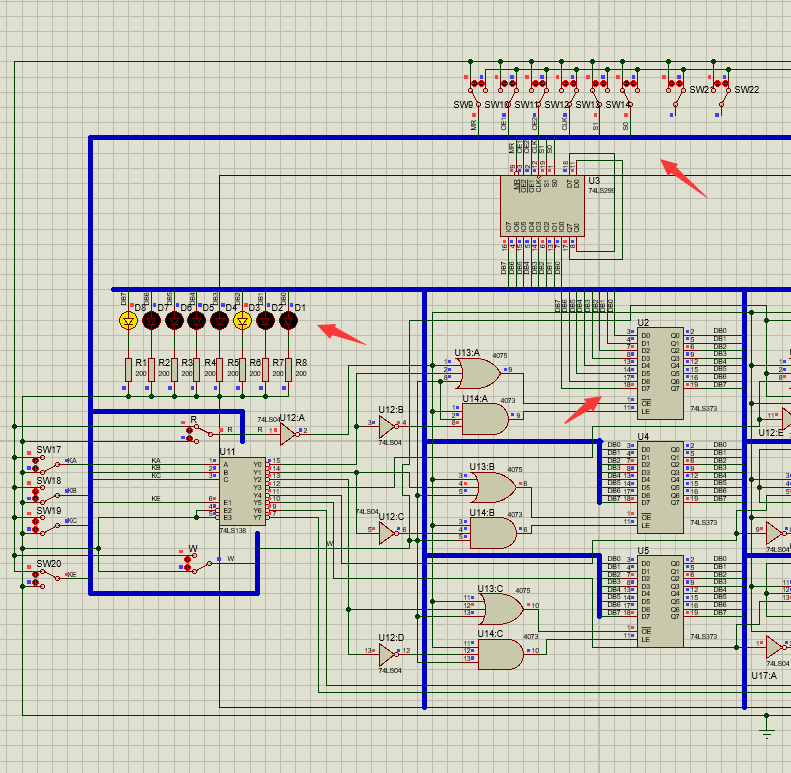思考问题

最后有一个小的思考问题 思考随着寄存器的增多，电路设计的复杂度是什么比例增大

寄存器增多，设计上来说复杂度是成倍的增加，电路的设计难度也有一定的提高。

原工程文件：
链接：https://pan.baidu.com/s/1mVnxtAznoFetXNdCohPYkg
提取码：hmcq

如果本文对你有小小的帮助，请点个赞再走吧 😄


展开全文74LS245
• 设计一个32×32位的寄存器堆（即含有32个寄存器，每个寄存器32位）
实验内容与原理
设计一个32×32位的寄存器堆（即含有32个寄存器，每个寄存器32位）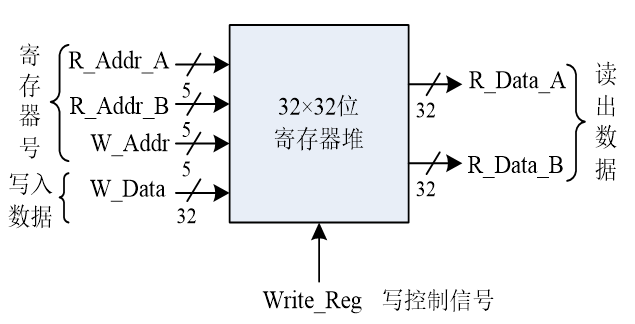实验实现：
寄存器堆：reg类型信号的数组 reg [31:0] REG_Files[0:31];
读操作：组合逻辑电路 assign R_Data_A = REG_Files[R_Addr_A]; assign R_Data_B = REG_Files[R_Addr_B];
写操作：时序逻辑电路 需要Reset信号：用于初始化寄存器（全部清零） 需要clk信号：用于写入寄存器
代码实现：
module register(
input [4:0]R_Addr_A,
input [4:0]R_Addr_B,
input [4:0]W_Addr,
input [31:0]W_Data,
input clk,
input rst,
input Write_Reg,
output [31:0]R_Data_A,
output [31:0]R_Data_B
);
reg [31:0] REG_Files[0:31];
integer i;
always @(posedge clk or posedge rst)
begin
if(rst) //高电平有效，=1则初始化
begin
for(i=0;i<32;i=i+1) REG_Files[i]<=0;
end
else
begin
if(Write_Reg)
REG_Files[W_Addr]<=W_Data;
end
end

//读数据
assign R_Data_A = REG_Files[R_Addr_A];
assign R_Data_B = REG_Files[R_Addr_B];

endmodule

测试代码：
module register_test;

// Inputs
reg [4:0] R_Addr_A;
reg [4:0] R_Addr_B;
reg [4:0] W_Addr;
reg [31:0] W_Data;
reg clk;
reg rst;
reg Write_Reg;

// Outputs
wire [31:0] R_Data_A;
wire [31:0] R_Data_B;

// Instantiate the Unit Under Test (UUT)
register uut (
.R_Addr_A(R_Addr_A),
.R_Addr_B(R_Addr_B),
.W_Addr(W_Addr),
.W_Data(W_Data),
.clk(clk),
.rst(rst),
.Write_Reg(Write_Reg),
.R_Data_A(R_Data_A),
.R_Data_B(R_Data_B)
);

always begin
#3 clk=~clk;
end
initial begin
// Initialize Inputs
R_Addr_A = 0;
R_Addr_B = 0;
W_Addr = 0;
W_Data = 0;
clk = 0;
rst = 1;
Write_Reg = 0;

// Wait 100 ns for global reset to finish
#2 rst=0;
#10
R_Addr_A = 5'b00001;
R_Addr_B = 5'b00110;
W_Addr = 5'b00001;
W_Data = 32'h00FE_8888;
Write_Reg = 1;
#10
R_Addr_A = 5'b00001;
R_Addr_B = 5'b00110;
W_Addr = 5'b00110;
W_Data = 32'h00FE_0EEE;
Write_Reg = 0;//测试写入信号为0的情况
#10 Write_Reg = 1;
#5
R_Addr_B = 5'b00111;
R_Addr_A = 5'b00110;
W_Addr = 5'b00010;
W_Data = 32'h00FE_1EEE;
#10
R_Addr_B = 5'b00010;
R_Addr_A = 5'b00110;
W_Addr = 5'b00011;
W_Data = 32'h00FE_2EEE;
#10
R_Addr_B = 5'b00010;
R_Addr_A = 5'b00011;
W_Addr = 5'b00100;
W_Data = 32'h00FE_3EEE;
#10
R_Addr_B = 5'b00100;
R_Addr_A = 5'b00011;
W_Addr = 5'b00101;
W_Data = 32'h00FE_4EEE;
#10
R_Addr_B = 5'b00100;
R_Addr_A = 5'b00101;
W_Addr = 5'b00110;
W_Data = 32'h00FE_5EEE;
#10
R_Addr_B = 5'b00100;
R_Addr_A = 5'b00110;
W_Addr = 5'b00111;
W_Data = 32'h00FE_6EEE;
#10
rst=1;

end

endmodule


波形：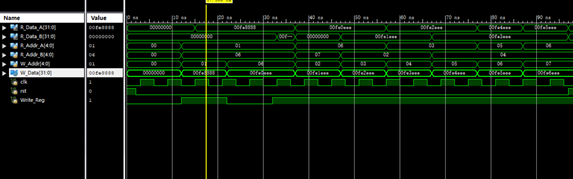利用ALU模块和本实验的寄存器堆模块，编写一个顶层模块，完成Ri θ Rj → Rk的操作（即2个寄存器数据做某种运算，结果送回第3个寄存器中）运算功能θ由ALU模块中的ALU_OP信号指定。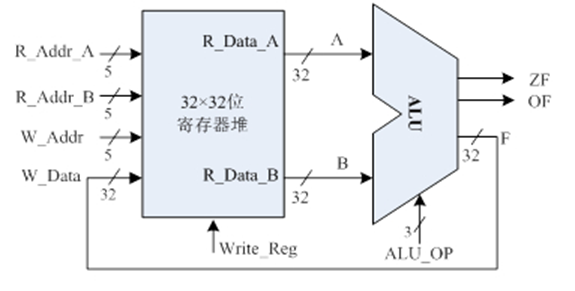实验代码
module ALU_register(
input [2:0]ALU_OP,
input [4:0]R_Addr_A,
input [4:0]R_Addr_B,
input [4:0]W_Addr,
input Write_Reg,
input clk,
input rst,
output ZF,
output OF,
output [31:0]R_Data_A,
output [31:0]R_Data_B,
output [31:0]F
);

register r1(
.R_Addr_A(R_Addr_A),
.R_Addr_B(R_Addr_B),
.W_Addr(W_Addr),
.Write_Reg(Write_Reg),
.W_Data(F),
.clk(clk),
.rst(rst),
.R_Data_A(R_Data_A),
.R_Data_B(R_Data_B)
);
ALU a(
.A(R_Data_A),
.B(R_Data_B),
.F(F),
.ZF(ZF),
.OF(OF),
.ALU_OP(ALU_OP)
);

endmodule


ALU代码见: 数字电路 多功能ALU设计实验.
测试代码（仅供参考）
module ALU_REG_test;

// Inputs
reg [2:0] ALU_OP;
reg [4:0] R_Addr_A;
reg [4:0] R_Addr_B;
reg [4:0] W_Addr;
reg Write_Reg;
reg clk;
reg rst;

// Outputs
wire ZF;
wire OF;
wire [31:0] R_Data_A;
wire [31:0] R_Data_B;
wire [31:0] F;

// Instantiate the Unit Under Test (UUT)
ALU_register uut (
.ALU_OP(ALU_OP),
.R_Addr_A(R_Addr_A),
.R_Addr_B(R_Addr_B),
.W_Addr(W_Addr),
.Write_Reg(Write_Reg),
.clk(clk),
.rst(rst),
.ZF(ZF),
.OF(OF),
.R_Data_A(R_Data_A),
.R_Data_B(R_Data_B),
.F(F)
);
always begin
#3 clk=~clk;
end
initial begin
// Initialize Inputs
ALU_OP = 0;
R_Addr_A = 0;
R_Addr_B = 0;
W_Addr = 0;
Write_Reg = 0;
clk = 0;
rst = 1;
#10
rst=0;
Write_Reg = 1;
R_Addr_A = 32'h0000_0000;
R_Addr_B = 32'h0000_0001;
W_Addr = 32'd0;
ALU_OP = 3'b011;
#10
Write_Reg = 1;
R_Addr_A = 32'h0000_0000;
R_Addr_B = 32'h0000_0001;
W_Addr = 32'd1;
ALU_OP = 3'b011;
#10

Write_Reg = 1;
R_Addr_A = 32'h0000_0000;
R_Addr_B = 32'h0000_0001;
W_Addr = 32'd10;
ALU_OP = 3'b000;
#3 R_Addr_A = 32'd10;//在每次写入之后都观察写入地址的数据，并且在时钟周期内所以不会写入
#10

Write_Reg = 1;
R_Addr_A = 32'h0000_0000;
R_Addr_B = 32'h0000_0001;
W_Addr = 32'd11;
ALU_OP = 3'b001;
#3 R_Addr_A = 32'd11;
#10

Write_Reg = 1;
R_Addr_A = 32'h0000_0000;
R_Addr_B = 32'h0000_0001;
W_Addr = 32'd12;
ALU_OP = 3'b010;
#3 R_Addr_A = 32'd12;
#10

Write_Reg = 1;
R_Addr_A = 32'h0000_0000;
R_Addr_B = 32'h0000_0001;
W_Addr = 32'd13;
ALU_OP = 3'b011;
#3 R_Addr_A = 32'd13;
#10

Write_Reg = 1;
R_Addr_A = 32'h0000_0000;
R_Addr_B = 32'h0000_0001;
W_Addr = 32'd14;
ALU_OP = 3'b100;
#3 R_Addr_A = 32'd14;
#10

Write_Reg = 1;
R_Addr_A = 32'h0000_0000;
R_Addr_B = 32'h0000_0001;
W_Addr = 32'd15;
ALU_OP = 3'b101;
#3 R_Addr_A = 32'd15;
#10

Write_Reg = 1;
R_Addr_A = 32'h0000_0000;
R_Addr_B = 32'h0000_0001;
W_Addr = 32'd16;
ALU_OP = 3'b110;
#3 R_Addr_A = 32'd16;
#10

Write_Reg = 1;
R_Addr_A = 32'h0000_0000;
R_Addr_B = 32'h0000_0001;
W_Addr = 32'd17;
ALU_OP = 3'b111;
#31 R_Addr_A = 32'd17;
#10

rst=1;

end

endmodule


波形图：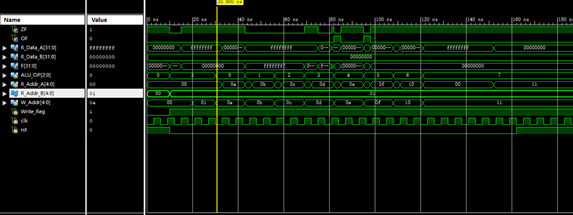展开全文• 计算机组成原理期末时交的课程设计 堆栈寄存器实验 自己做的不是太好 希望有点帮助
• 寄存器是中央处理器内的组成部分 寄存器是有限存贮容量的高速存贮部件 用来暂存指令、数据和地址 MIPS指令需要32个寄存器，采用寄存器-寄存器结构 需要用数组表示寄存器寄存器堆需要有两个数据输出接口，同时输出...
寄存器
寄存器是中央处理器内的组成部分
寄存器是有限存贮容量的高速存贮部件
用来暂存指令、数据和地址
寄存器堆
MIPS指令需要32个寄存器，采用寄存器-寄存器结构
需要用数组表示寄存器堆
寄存器堆需要有两个数据输出接口，同时输出；一个输入接口
0号寄存器不保存数据，固定为0【用来完成一些简单指令
读
raddr1读地址
若raddr=00111，则rdata1写入7号寄存器的数据
写四个要求
1 waddr哪个寄存器
2 wdata数据
3 clk等脉冲上升沿
4 WE读写信号高电平
寄存器堆接口
module registers（
input clk,
input oc,	//可不要
input [4:0] raddr1,
input [4:0] raddr2,
input [4:0] waddr,
input [31:0] wdata,
input we,	//使能端，读写信号
output reg[31:0] rdata1,
output reg[31:0] rdata2
);
reg [31:0] rdata1;
reg [31:0] rdata2;
reg [31:0] regts[1:31];
//读端口1读端口2用两个独立的always模块来实现
//读端口1
always @(*)	//组合逻辑电路，只要有输入马上就有输出
begin
if(oc==1'b1)	//禁止输出
begin
radata1<=32'b0;
end
else if(raddr1==5'b00000)	//$0号寄存器只保存0 begin rdata1<=32'b0; end else begin rdata1<=regts[raddr1] end end //读端口2 //写端口 always @（posedge clk) //脉冲信号作用下才能写 begin if((we==1'b1)&&(waddr!=5'b00000)) //判断使能端是否为1，是否为0号地址，0号不可写 begin regts[waddr]<=wdata; end end  ALU&&寄存器堆 寄存器堆rdata1接ALU a端口，寄存器堆rdata2接ALU b端口 ALU输出f端口接寄存器堆wdata端口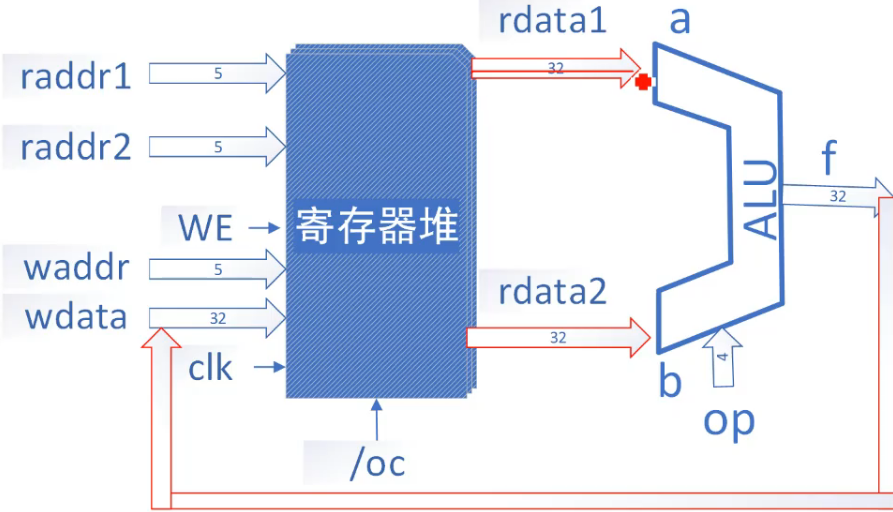reg(…out)输出端口与alu(a…)输入端口位数一致 wire[3:0]w, //声明wire电线连接，两两之间 reg r(…w), alu a(w…), fib整体框架图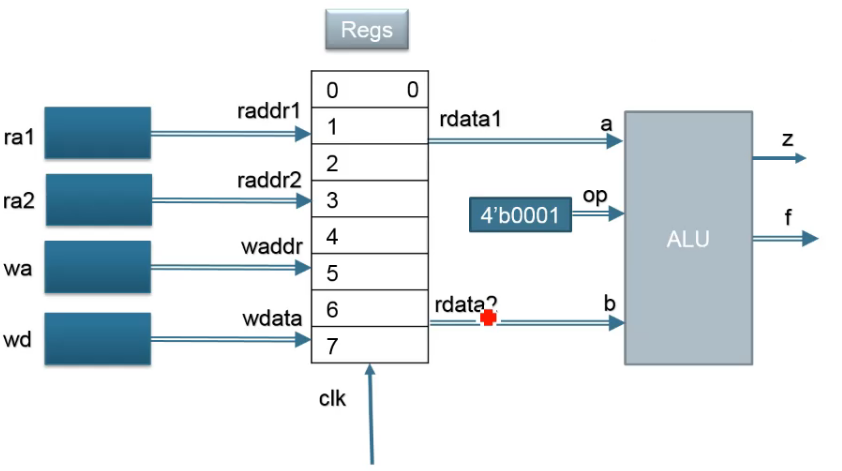操作确定：加法 默认：WE=1 初始除了0号寄存器其他寄存器均为空 通过写端口写入数据 rada1 rdata2数据来自寄存器 有限状态机fsm always@(posedge clk)//时钟脉冲的作用下 if(reset)//复位信号有效，直接跳转到初始状况 … else case(state) STATE1: … //指出每个状态执行完跳转到哪个状态 STATE1: … … default:state <= STATE1; endcase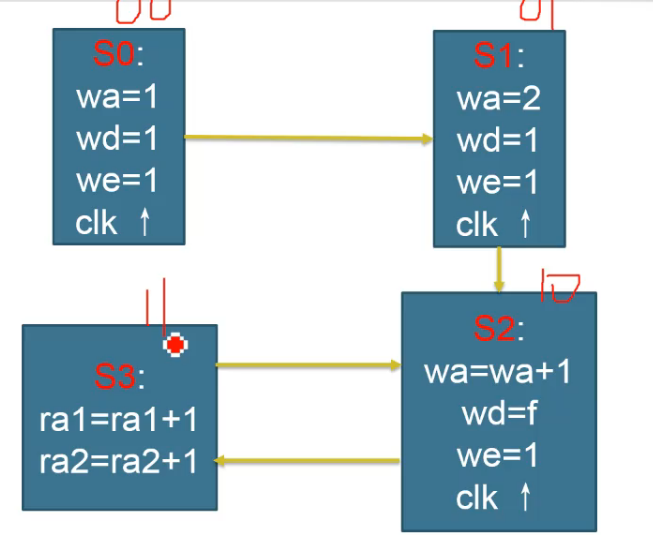fib&fsm //申请状态 wire [31:0] a, b ,f; reg [31:0] wdata; wire we = 1'b1; parameter op = 4'b0001; //加法运算 reg [4:0] ra1,ra2,wa; reg [3:0] count; //实例化寄存器 registers myregs(.clk(clk),.oc(~rst),.raddr1(ra1),.raddr2(ra2),.waddr(wa),.wda(wdata),.we(we),.rdata1(a),.rdata2(b)); //实例化ALU alu myalu(a, b, op, f); //a b与rdata1(a),rdata2(b)连接 assign result = wdata; reg[1:0]status = 2'b00; //状态 always@(posedge clk) begin if(rst == 1'b0) //复位信号 begin status <= 2'b00; //初始化为0 count <= 3; //从3开始算 end else case(status) 2’b00: begin ra1 <= 5'b1; ra2 <= 5'b10; wa <= 5'b1; //1号寄存器 wdata <= 32'b1; //1写入1号寄存器 status <= 2'b01; //跳转到下一状态 end 2’b01: begin wa <= 5'b10; wdata <= 32'b1; status <= 2'b10; end 2'b10: begin wa <= ra2+1; wdata <= f; status <= 2'b11; count <= count + 1; end 2'b11: begin if(count < n) begin ra1 <= ra2; //ra1=ra1+1 ra2 <= wa; //ra2=ra2+1 status <= 2'b10; end end endcase  最后补充-所有模块代码 //top模块： module top2( input clk, input rst, input [3:0] n, output [11:0] result ); wire [31:0] out; assign result=out[11:0]; fib1 f(clk,rst,n,out); initial$monitor($time,,"top:result=%h",result); endmodule // //fib模块： module fib1( input clk, input rst, input [3:0] n, output [31:0] result ); wire [31:0] a,b,f; reg [31:0] wdata; wire we = 1'b1; reg [4:0] ra1,ra2,wa; reg [3:0] count; parameter op = 4'b0001; //加法运算 //实例化寄存器 registers myregs(.clk(clk),.oc(~rst),.raddr1(ra1),.raddr2(ra2),.waddr(wa),.wdata(wdata),.we(we),.rdata1(a),.rdata2(b)); //实例化ALU alu myalu1(a, b, op, f); //a b与rdata1(a),rdata2(b)连接 assign result = wdata; reg[1:0]status = 2'b00; //状态 always@(posedge clk) begin if(rst == 1'b0) //复位信号 begin status <= 2'b00; //初始化为0 count <= 3; //从3开始算 end else case(status) 2'b00: begin ra1 <= 5'b1; ra2 <= 5'b10; wa <= 5'b1; //1号寄存器 wdata <= 32'b1; //1写入1号寄存器 status <= 2'b01; //跳转到下一状态 end 2'b01: begin wa <= 5'b10; wdata <= 32'b1; status <= 2'b10; end 2'b10: begin wa <= ra2+1; wdata <= f; status <= 2'b11; count <= count + 1; end 2'b11: begin if(count < n) begin ra1 <= ra2; //ra1=ra1+1 ra2 <= wa; //ra2=ra2+1 status <= 2'b10; end end endcase end initial$monitor($time,,"fib:rst=%b,wdata=%h,count=%h",rst,wdata,count); initial$monitor($time,,"fib:result=%h",result); endmodule // //alu模块： module alu( input [31:0] a, input [31:0] b, input [3:0] operation, output [31:0] f, output z ); reg[31:0]result; always@(*) begin case(operation) 4'b0000:result=32'b0; 4'b0001:result=a+b; 4'b0010:result=a-b; 4'b0011:result=a&b; 4'b0100:result=a|b; 4'b0101:result=a^b; default:result=32'b0; endcase end assign f=result; assign z=~(|result); initial$monitor($time,,"alu:a=%h,b=%h,operation=%b",a,b,operation); initial$monitor($time,,"alu:f=%h,z=%b",f,z); endmodule // //registers模块： module registers( input clk, input oc, //可不要 input [4:0] raddr1, input [4:0] raddr2, input [4:0] waddr, input [31:0] wdata, input we, //使能端，读写信号 output [31:0] rdata1, output [31:0] rdata2 ); reg [31:0] rdata1; reg [31:0] rdata2; reg [31:0] regts[1:31]; //读端口1 always @(*) //只要有输入就立即输出 begin if(oc == 1'b1) //禁止输出 begin rdata1 <= 32'b0; end else if(raddr1 == 5'b00000) //$0号寄存器只保存0
begin
rdata1 <= 32'b0;
end
begin
rdata1 <= regts[raddr1];
end
end
//读端口2
always @(*)
begin
if(oc == 1'b1)
begin
rdata2 <= 32'b0;
end
else if(raddr2 == 5'b00000)
begin
rdata2 <= 32'b0;
end
begin
rdata2 <= regts[raddr2];
end
end

always @(posedge clk)		//脉冲信号作用下才能写
begin
#1 if((we == 1'b1) &&(waddr != 5'b00000))		//判断使能端是否为1，是否为0号地址，0号寄存器不可写
begin
regts[waddr] <= wdata;
end
end
endmodule

//仿真程序
module fib_sim(
output [11:0] result
);
//parameter clk_period=10;
reg clk;
initial
begin
clk=1'b0;
forever
#2 clk=~clk;
end

reg rst=1'b1;
reg[3:0] n=4'b1111;
top2 t(clk,rst,n,result);

initial
begin
#2 rst=1'b0;
#4 rst=1'b1;
end

endmodule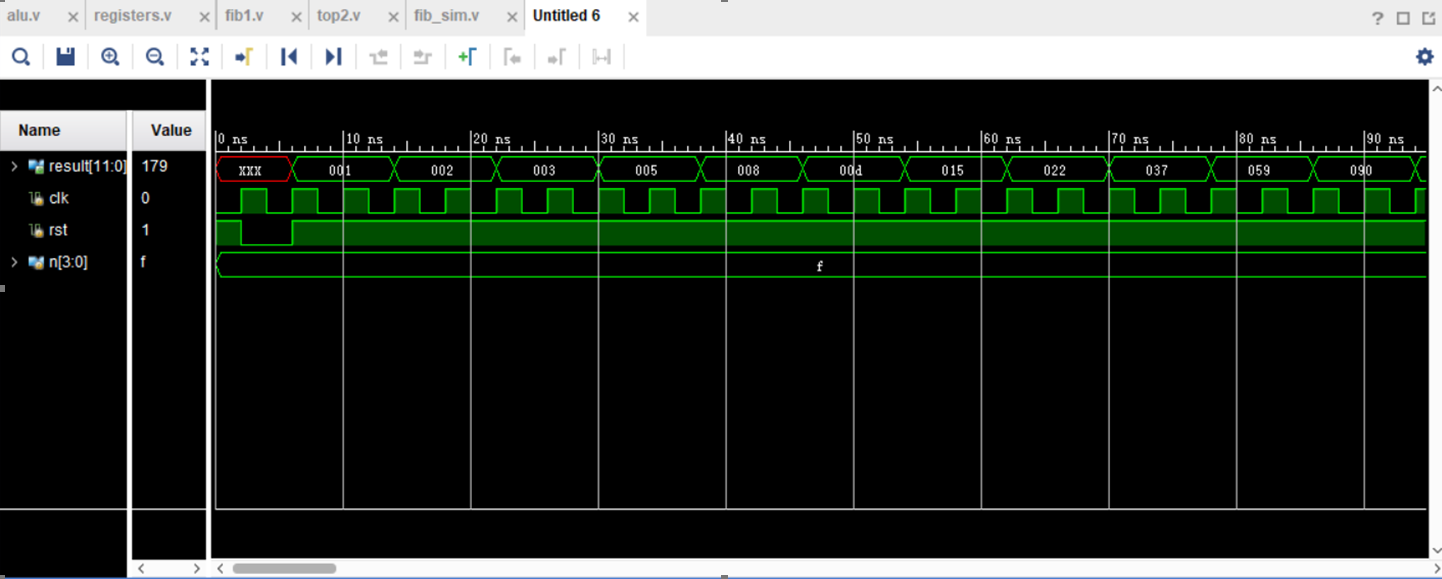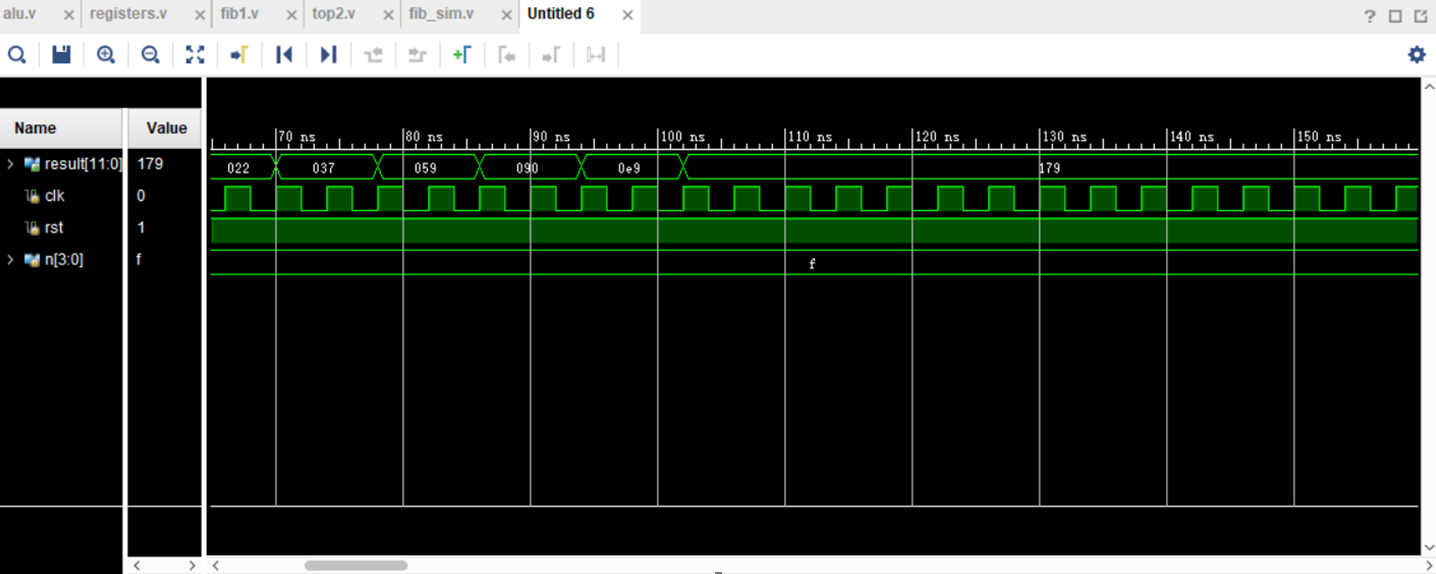仿真到179’h即停止的原因是输入n的位数为4位。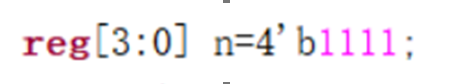展开全文fpga
• 寄存器是CPU中的一个重要组成部分，它是CPU内部的临时存储单元。寄存器既可以用来存放数据和地址，也可以存放控制信息或CPU工作时的状态。在CPU中增加寄存器的数量，可以使CPU把执行程序时所需的数据尽可能地放在...
寄存器是CPU中的一个重要组成部分，它是CPU内部的临时存储单元。寄存器既可以用来存放数据和地址，也可以存放控制信息或CPU工作时的状态。在CPU中增加寄存器的数量，可以使CPU把执行程序时所需的数据尽可能地放在寄存器件中，从而减少访问内存的次数，提高其运行速度。但是，寄存器的数目也不能太多，除了增加成本外，由于寄存器地址编码增加也会相对增加指令的长度。CPU中的寄存器通常分为存放数据的寄存器、存放地址的寄存器、存放控制信息的寄存器、存放状态信息的寄存器和其他寄存器等类型。

程序状态寄存器用于记录运算中产生的标志信息，典型的标志为有进位标志位、零标志位、符号标志位、溢出标志位和奇偶标志等。状态寄存器中的各个状态标志位是依据算术逻辑部件上次的运算结果来置位的。

地址寄存器包括程序计数器、堆栈指示器、变址寄存器和段地址寄存器等，用于记录各种内存地址。

程序计数器用于存放指令的地址。当程序顺序执行时，每取出一条指令，PC内容自动增加一个值，指向下一条要取的指令。当程序出现转移时，则将转移地址送入PC，然后由PC指向新的程序地址。程序计数器PC中存放的是转移的目标地址。

累加寄存器是一个数据寄存器，在运算过程中暂时存放被操作数和中间运算结果，累加器不能用于长时间地保存一个数据。

1、CPU中有若干寄存器，保存当前正在执行的指令的寄存器为指令寄存器IR，保存下一条指令地址的寄存器为程序计数器PC，用于存放存储器中数据和指令地址的寄存器是地址寄存器，存数据和指令的寄存器是数据寄存器。

2、通用寄存器可用于传送和暂存数据，也可参与算术逻辑运算，并保存运算结果。除此之外，它们还各自具有一些特殊功能。通用寄存器的长度取决于机器字长，汇编语言程序员必须熟悉每个寄存器的一般用途和特殊用途，只有这样，才能在程序中做到正确、合理地使用它们。

3、指令寄存器(IR)用来保存当前正在执行的一条指令，不需要用户的任何干预，所以对用户是透明的。当执行一条指令时，先把它从内存取到数据寄存器(DR)中，然后再传送至IR。指令划分为操作码和地址码字段，由二进制数字组成。为了执行任何给定的指令，必须对操作码进行测试，以便识别所要求的操作。指令译码器就是做这项工作的。指令寄存器中操作码字段的输出就是指令译码器的输入。操作码一经译码后，即可向操作控制器发出具体操作的特定信号。

4、操作数在寄存器中的寻址方式为“寄存器直接寻址”，也叫“寄存器寻址”。当操作数的内存偏移地址在寄存器中时叫“寄存器间接寻址”。

5、CPU内通用寄存器的位数与机器字节有关。

通常一个寄存器保存一个机器字长的数据，通用寄存器的最大位数等于机器字长。

6、主存地址寄存器MAR的位数与程序计数器PC寄存器相同，都取决于主存储器的容量。

7、汇编程序员可以通过指定待执行指令的地址来设置程序计数器（PC）的值，也可用通用寄存器（GR），而IR（指令寄存器）、MAR（存储地址寄存器）、MDR（存储器数据寄存器）是CPU的内部工作寄存器，对程序员不可见。

8、在键盘接口中设置移位寄存器是为了实现串-并转化。

键盘的输入是一位进行的，但传入主存的信息是并行的，所以，键盘接口必须实现串行到并行的转换。

9、状态寄存器用来存放算术、逻辑运算及测试指令的结果状态。

10、在CPU中，累加寄存器可用于传送和暂存用户数据，为ALU执行算术逻辑运算提供工作区。

展开全文• 实验内容： 1. 将58H写入A寄存器。 2. 将6BH写入W寄存器。 3. 将ACH写入R2寄存器。 ...这些寄存器包括累加器A，工作寄存器W，数据寄存器组R0…R3，地址寄存器MAR，堆栈寄存器ST，输出寄存器OUT等。
• 这次要做的是用Verilog代码写一个寄存器堆，此寄存器堆共有32个寄存器，每个寄存器可存储32个二进制位。要求有一个写端口，两个读端口，本次实验设计为异步读同步写的寄存器堆，即读寄存器不需要时钟控制，但写...Verilog Vivado
• proteus仿真软件安装教程链接 如果对我的实验报告感兴趣，可以考虑我的计组实验专栏 计算机组成原理专栏链接
• 计算机组成原理上机报告，Verilog语言实现8位移位寄存器，并且仿真波形。实验环境为Quartus II，编程语言Verilog，文档排版使用LaTeX，内附LaTeX源文件，可修改。
• 第一部分 移位寄存器实验 一、实验目的 验证移位寄存器的组合功能。 二、实验内容 1、实验原理 移位运算实验原理如图1.1所示。移位运算实验原理如图3－4所示，使用了一片74LS299（U34）作为...
• 东北大学软件学院，计算机组成原理实验--通用寄存器的实现。
• 这些寄存器用来暂存一个计算机字。根据需要，可以扩充其数目。下面详细介绍这些寄存器的功能与结构。 1.数据缓冲寄存器（DR） 数据缓冲寄存器用来暂时存放由内存储器读出的一条指令或一个数据字；反之，当向内存...
• 结合做了一些小修改，使文章更完整。 1 总线 只是想了解寄存器知识的话，只要看这一段就好...计算机五大组成部分是：控制器、运算器、存储器、输入设备和输出设备。CPU所代表的控制器和运算器需要和存储器（主内存），cpu
• 计算机组成原理实验报告-存储器,其中包含实验内同,实验目的,实验结果还有电路图
• 文件为 jed sch syn 格式文件 所用系统是白中英教材配套的实验系统
• 常见的寻址方式有立即寻址、直接寻址、间接寻址、寄存器寻址、寄存器间接寻址、相对寻址和变址寻址等。在立即寻址方式中，操作数包含在指令中；在直接寻址方式中，操作数存放在内存单元中；在寄存器寻址方式中，操作...
• 使用Logisim设计和实现mips寄存器堆（即，regFile），具体要求如下： 1，包含两个读寄存器号输入端口RD1和RD2，用来分别指定待读出数据的两个寄存器，这两个寄存器可能相同，也可能不同。读出的数据分别通过data1和...嵌入式 python 物联网 fpga 算法
• 程序计数器PC的位数 ...指令寄存器的位数 由指令长度决定，而指令又有半字长、单子长、双字长、多字长的长度类型，所以长度不确定。（这里的字长是机器字长）。 通用寄存器的位数 取决于机器字长的位数。 ...
• 实验一 寄存器A，W实验 一、实验要求 利用COP2000实验仪上的K16..K23开关做为DBUS的数据，其它开关做为控制信号，将数据写入寄存器，这些寄存器包括累加器A，...了解模型机中A和W寄存器结构、工作原理及其控制方法。
• 实验内容与原理: CPU内部通常包含若干个通用寄存器，以暂存参加运算的数据和中间结果。寄存器速度快，个数少，但是RISC CPU的设计强调设置大量的寄存器。例如，Intel 80x86系列CPU中只有8个寄存器，而SPARC RISC ...ALU运算器
• 计算机组成原理学习-实验二 （详细、系统） 在线博主，及时解答~嵌入式 经验分享 后端 程序人生
• 1.PC和MAR寄存器的位数对应的是存储单元的个数 eg:若MAR为10位,则对应2**10=1024个存储单元,记为1K.也即像2M,1K,3G等表示的都是存储单元的个数.当其后加上B表示的是字节个数.例如:2MB表示2M个字节.
• 寄存器的内部结构：2.D触发器：1.触发器基本特征:2.工作原理：3.两台相连的D触发器：4.示例图：3.寄存器的构成： 1.寄存器的内部结构： 2.D触发器： 1.触发器基本特征: 2.工作原理： 将左边的显示器与输入端口D...
• CPU，主要有算术和逻辑运算单元ALU，地址发生和控制单元，指令译码单元数据寄存器单元，总线驱动单元，时序控制单元等组成。 存储器，就是分为内存外存，在此不多赘述。 输入输出设备，非常常见，典型的显示屏，键盘...编程语言
• 寄存器堆实验实验原理实验内容模块功能说明模块逻辑引脚图寄存器堆模块代码REG模块测试用例REG-ALU测试用例实验结果记录 寄存器堆实验结果及分析ALU_REG运算器模块实验结果及分析探索与思考 实验原理   32×32位......